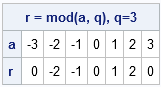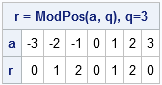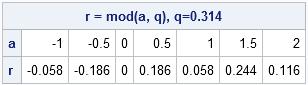When I studied math in school, I learned that the expression a (mod n) is always an integer between 0 and q – 1 for integer values of a and q.

It's a nice convention, but SAS and many other computer languages allow the result to be negative if a (or q) is negative. That is, if you write r = mod(a, q), then all you can say is that the ABSOLUTE VALUE of r is between 0 and q – 1. The documentation of the MOD function in SAS says:

The MOD function returns the remainder from the division of a by q. When the result is non-zero, the result has the same sign as the first argument. The sign of the second argument is ignored.

This fact "bit me" the other day when I was writing a function that used the MOD function. I had assumed that the remainder would always be positive, and this led to a runtime error when I was testing my program. Statistical programmers who program in multiple languages should be aware that modulus function (or operator) in MATLAB and R have a different behavior: both languages ignore the sign of the first argument and use the sign of the second argument.

The following SAS/IML program shows the SAS behavior:

```proc iml; q = 3; a = -q:q; r = mod(a, q); print (a // r)[r={"a" "r"} label="r = mod(a, q), q=3"];```If you always want the result to be positive, it is easy to define your own function in SAS/IML (or by using PROC FCMP):

```start ModPos(a, q); return( a - q*floor(a/q) ); finish;   r2 = ModPos(a,q); print (a // r2)[r={"a" "r"} label="r = ModPos(a, q), q=3"];```While I was reading the documentation for the MOD function, I also realized that the MOD function supports arguments that are not integers, such as the following example:

```q = 0.314; a = do(-1, 2, 0.5); r3 = mod(a, q); print (a // r3)[r={"a" "r"} label="r = mod(a, q), q=0.314"];```I'm not sure why I would use this feature, but I'll keep it in mind in case I should need it someday.

ShareDistinguished Researcher in Computational Statistics

Rick Wicklin, PhD, is a distinguished researcher in computational statistics at SAS and is a principal developer of SAS/IML software. His areas of expertise include computational statistics, simulation, statistical graphics, and modern methods in statistical data analysis. Rick is author of the books Statistical Programming with SAS/IML Software and Simulating Data with SAS.

1.I ran into this exact problem just yesterday; my solution was the following:
r=mod(mod(a,q)+q,q)

2.Any particular reason why you do not use Abs, when you want the value to be positive?
Either on the input side, or the output side?

•The expression r3 = mod(abs(a), q) is not equivalent to r2, so it's not the answer that I want.

•Ah, I see it now.
You want the pattern (0, 1, 2, 0, 1, 2, 0 ... for q=3) to be continuous before and after 0, whereas the pattern created by the remainder of the division will flip the pattern and sign around 0.

3.I want the result of mod(a,q) to be in the range {0,1,...,q-1}. Neither SAS mod nor your PosMod will do: PosMod(2,3) = -1.

•For positive arguments, mod(a,q) is always in the range {0, 1, ..., q-1}. For a=2 and q=3, mod(2,3)=2.